# SAT II Physics : Optics

## Example Questions

### Example Question #1 : Optics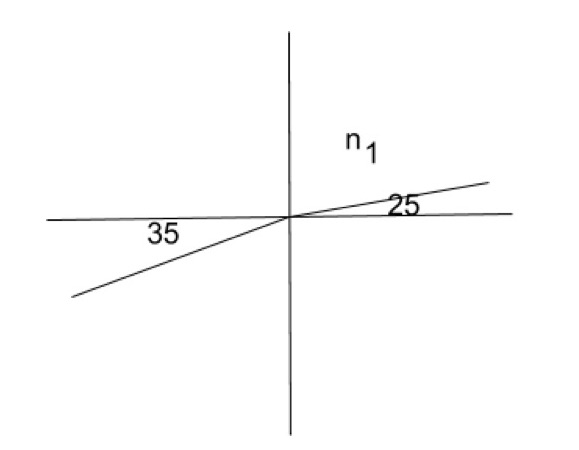Light passes from a medium with some index of refraction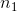at 25 degrees from perpendicular and exits into another medium with an index of refraction of 1.5 at an angle of 35 degrees. What is the value of?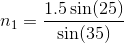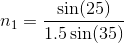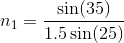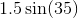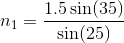Explanation:

Snell's law states: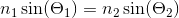If we substitute the values from the question we get: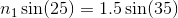Next rearrange to isolate### Example Question #1 : Optics, Waves, And Quantum

Which of the following scenarios would result in the polarization of light?

Light is reflected off of a glass window

Light passes through a vacuum

Light pass between two mediums with the same index of refraction

A light source travels at a high velocity

Light is absorbed by a black body

Light is reflected off of a glass window

Explanation:

Light is polarized when it is all oscillating in the same direction. This effect occurs when light is reflected. Polarization can occur through refraction, however if light is passing between mediums with the same index of refraction, the light will not refract.

### Example Question #1 : Optics

Of the following, which type of waves has the longest wavelength?

Microwaves

Gamma rays

Orange light

Green light

X-rays